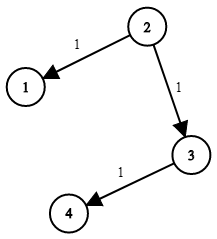Formatted question description: https://leetcode.ca/all/743.html

# 743. Network Delay Time (Medium)

There are N network nodes, labelled 1 to N.

Given times, a list of travel times as directed edges times[i] = (u, v, w), where u is the source node, v is the target node, and w is the time it takes for a signal to travel from source to target.

Now, we send a signal from a certain node K. How long will it take for all nodes to receive the signal? If it is impossible, return -1.

Example 1:Input: times = [[2,1,1],[2,3,1],[3,4,1]], N = 4, K = 2
Output: 2


Note:

1. N will be in the range [1, 100].
2. K will be in the range [1, N].
3. The length of times will be in the range [1, 6000].
4. All edges times[i] = (u, v, w) will have 1 <= u, v <= N and 0 <= w <= 100.

Related Topics:
Heap, Depth-first Search, Breadth-first Search, Graph

## Solution 1. Dijkstra

// OJ: https://leetcode.com/problems/network-delay-time/
// Time: O(E + VlogV)
// Space: O(E)
class Solution {
typedef unordered_map<int, unordered_map<int, int>> Graph;
typedef pair<int, int> iPair;
vector<int> dijkstra(Graph graph, int N, int source) {
priority_queue<iPair, vector<iPair>, greater<iPair>> pq;
vector<int> dists(N, INT_MAX);
pq.emplace(0, source);
dists[source] = 0;
while (pq.size()) {
int u = pq.top().second;
pq.pop();
for (auto neighbor : graph[u]) {
int v = neighbor.first, weight = neighbor.second;
if (dists[v] > dists[u] + weight) {
dists[v] = dists[u] + weight;
pq.emplace(dists[v], v);
}
}
}
return dists;
}
public:
int networkDelayTime(vector<vector<int>>& times, int N, int K) {
Graph graph;
for (auto e : times) graph[e - 1][e - 1] = e;
auto dists = dijkstra(graph, N, K - 1);
int ans = 0;
for (int d : dists) {
if (d == INT_MAX) return -1;
ans = max(ans, d);
}
return ans;
}
};


## Solution 2. Bellman-Ford

// OJ: https://leetcode.com/problems/network-delay-time/
// Time: O(VE)
// Space: O(V)
class Solution {
vector<int> bellmanFord(vector<vector<int>>& edges, int V, int src) {
vector<int> dist(V, INT_MAX);
dist[src - 1] = 0;
for (int i = 1; i < V; ++i) {
for (auto &e : edges) {
int u = e - 1, v = e - 1, w = e;
if (dist[u] == INT_MAX) continue;
dist[v] = min(dist[v], dist[u] + w);
}
}
return dist;
}
public:
int networkDelayTime(vector<vector<int>>& times, int N, int K) {
auto dist = bellmanFord(times, N, K);
int ans = *max_element(dist.begin(), dist.end());
return ans == INT_MAX ? -1 : ans;
}
};


Java

• class Solution {
public int networkDelayTime(int[][] times, int N, int K) {
Map<Integer, List<int[]>> travelMap = new HashMap<Integer, List<int[]>>();
for (int[] time : times) {
int source = time - 1, target = time - 1, lapse = time;
List<int[]> travels = travelMap.getOrDefault(source, new ArrayList<int[]>());
travelMap.put(source, travels);
}
for (int i = 0; i < N; i++)
queue.offer(new int[]{K - 1, 0});
while (!queue.isEmpty()) {
int[] nodeTime = queue.poll();
int node = nodeTime, time = nodeTime;
List<int[]> travels = travelMap.getOrDefault(node, new ArrayList<int[]>());
for (int[] travel : travels) {
int target = travel, lapse = travel;
int totalLapse = time + lapse;
queue.offer(new int[]{target, totalLapse});
}
}
}
int maxTime = 0;
for (int time : received) {
if (time == Integer.MAX_VALUE)
return -1;
else
maxTime = Math.max(maxTime, time);
}
return maxTime;
}
}

• // OJ: https://leetcode.com/problems/network-delay-time/
// Time: O(E + VlogV)
// Space: O(E)
class Solution {
typedef pair<int, int> PII;
public:
int networkDelayTime(vector<vector<int>>& E, int n, int k) {
vector<vector<PII>> G(n);
for (auto &e : E) G[e - 1].emplace_back(e - 1, e);
vector<int> dist(n, INT_MAX);
dist[k - 1] = 0;
priority_queue<PII, vector<PII>, greater<>> pq;
pq.emplace(0, k - 1);
while (pq.size()) {
auto [cost, u] = pq.top();
pq.pop();
if (dist[u] > cost) continue;
for (auto &[v, w] : G[u]) {
if (dist[v] > dist[u] + w) {
dist[v] = dist[u] + w;
pq.emplace(dist[v], v);
}
}
}
int ans = *max_element(begin(dist), end(dist));
return ans == INT_MAX ? -1 : ans;
}
};

• class Solution:
def networkDelayTime(self, times, N, K):
"""
:type times: List[List[int]]
:type N: int
:type K: int
:rtype: int
"""
K -= 1
nodes = collections.defaultdict(list)
for u, v, w in times:
nodes[u - 1].append((v - 1, w))
dist = [float('inf')] * N
dist[K] = 0
done = set()
for _ in range(N):
smallest = min((d, i) for (i, d) in enumerate(dist) if i not in done)
for v, w in nodes[smallest]:
if v not in done and dist[smallest] + w < dist[v]:
dist[v] = dist[smallest] + w